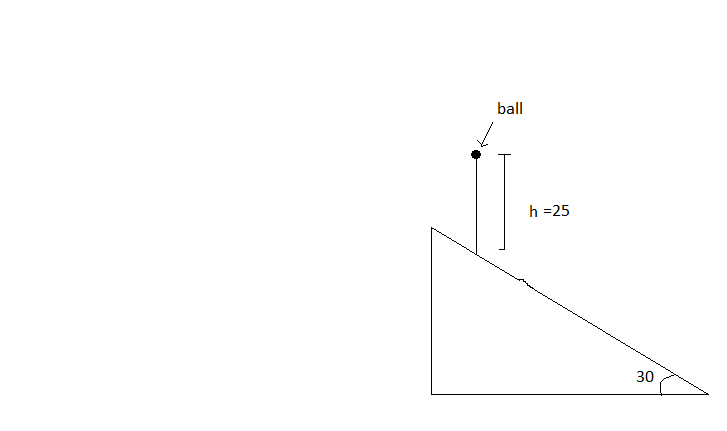# 200 followers problemIf distance between the two points on the fixed smooth inclined plane on which the ball makes first and second collision can be represented as $\Delta$.

Find $\sqrt{\Delta}$.

Details

• Collisions are perfectly elastic. -surface of inclined plane is smooth.
• No air resistance.
• $h=25m,B=30^{\circ}$
×